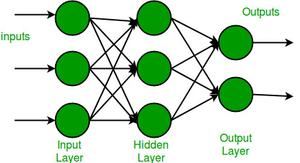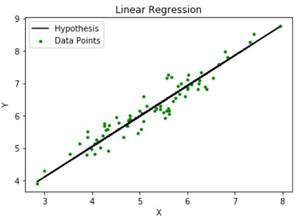# Difference between Multilayer Perceptron and Linear Regression

• Last Updated : 07 Mar, 2022

Multi-layer perceptrons (MLP) is an artificial neural network that has 3 or more layers of perceptrons. These layers are- a single input layer, 1 or more hidden layers, and a single output layer of perceptrons. The data flows in a single direction, that is forward, from the input layers-> hidden layer(s) -> output layer. Backpropagation is a technique where the multi-layer perceptron receives feedback on the error in its results and the MLP adjusts its weights accordingly to make more accurate predictions in the future. MLP is used in many machine learning techniques like classification and regression. They have been shown to give highly accurate results for classification problems in particular.

Regression is a supervised machine learning technique that approximates a continuous-valued variable. It is generally used to forecast/predict values based on the values of the independent variable. A regression problem can be thought of as having a single output neuron with no activation function.Multi-layer perceptronsLinear Regression Graph

These are the differences between multi-layer perceptrons and linear regression-

My Personal Notes arrow_drop_up